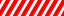Quadratic Formula Calculator will help you to solve the quadratic equations online. Just enter a, b, and c values and get the quick results. Also, you can find it’s a formula in this article.

 A = B = C = The Quadratic Equation: x2 + x + = 0Discriminant: Δ  = Quadratic Formula: x1,2= First Root: x1   = Second Root: x2 =
Related

In mathematics, the most interesting equation we come across is the Quadratic Equations. In our school, we were taught formulas for finding the roots of quadratic equations.We always wished that if there was a Quadratic Formula Calculator which would make our work easier.

Now, this thing is possible, and no need to go through the long boring procedure.

Calculating the roots of the second-order polynomial equations or quadratic equations is very simple using this Equation Solver.

The standard form of a quadratic equation is:

#### ax2 + bx + c = 0

It has two roots. Solving a quadratic equation that means finding the roots. Sometimes at first complex equations are used to make it in standard form. Then values of A, B, and C are used in the formula to get the roots.

The formula for finding the roots is:The term b2 – 4ac is known as Discriminant. Two roots(x1 and x2) are calculated once by putting the positive sign and another one by putting a negative sign.There are three possibilities of getting the types of roots. It depends upon the value of the discriminant.

• If b2 – 4ac = 0 then there will be only one real root.
• If b2 – 4ac > 0 then there will are two real roots.
• And if b2 – 4ac < 0 then there will be two complex roots.

## How Quadratic Formula Calculator Works?

The process used behind the quadratic formula calculator is the same as mathematics equations. Just you don’t need to do the manual calculation during the process. Only you are required to put the coefficients. After that press the “Calculate” button to get answer.

For example, your quadratic equation is 4x2 – 2x + 1 = 0. Therefore, your coefficients are:

a = 4
b = -2
c = 1

So, you need to enter them in the input boxes. Remember a is the coefficient of the squared variable, b is for one – degree variable, and c is the last one.

Meanwhile, let’s solve the above equation using the root formula. The most important thing is to know the coefficients.

Now put these values in the formula. After that solve it once by taking a positive sign and another time by taking a negative sign to get the two roots.

Find the Discriminant ( b2 – 4ac ). Then check the conditions of the roots. The final roots of the quadratic equation will be:

X1 = 0.25 + 0.433013i
X2 = 0.25 – 0.433013i

You can use the Quadratic Formula Calculator to do this calculation online. It is the best tool for finding the roots of any equation.

#### Why use Quadratic Equation Calculator?

1. Manual calculations take very much time. Subsequently, it becomes worst when there are some complex equations. Therefore, to save time and effort we should avoid manual calculations. On the other hand, using this tool you can easily get results in seconds.
2. No one can guarantee that the human brain will not commit a mistake. However, in the case of computers, the chances of errors are nearly zero. The tool is pre-programmed, and all the time works using the same algorithms.
3. Availability is another most important factor. Generally, we carry our smartphones and other devices everywhere. In many cases, it happens that you don’t have a pen and paper to solve the equation. In that case, this tool is very helpful. Therefore, you should always consider using an online quadratic formula calculator.

#### How to Use Quadratic Formula Calculator?

The Quadratic Equation Solver is an online service. Hence, it is only working if you have an Internet connection. Also, you should have a device that can access the Internet. Once you are fulfilled above all these requirements just open your browser and open the Quadratic Formula Calculator.

As mentioned above you should first arrange the coefficients and then enter them in proper input boxes. But if the equation is not in the standard form then simplify it and make it in standard form. Moreover, remember to check the signs(+/-) of the coefficients. After entering values press the “Calculate” button. As a result, you will get discriminant, quadratic formulas, and roots.